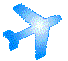Aerodynamics Terms and Definitions
Вие се намирате тук: Начална страница > Информационен справочник > Авиационни терминологични речници > Aerodynamics Terms and Definitions

Aerodynamic Center
The aerodynamic center is a point along the airfoil or wing about which the moment coefficient does not vary with an angle of attack change.

Airfoil
An airfoil is the cross section of a wing. The airfoil shape and variations in angle of attack are primarily responsible for the lift and profile drag of the wing.

Angle of Attack
The angle of attack is defined as the angle between the plane of the wing (airfoil chord) and the direction of motion (free stream velocity). The angle of attack can be varied to increase or decrease the lift acting on the wing. An increase in lift often results in an increase in drag.

Center of Pressure
A point along the airfoil about which the moment due to the lift is zero, i.e., it is the point of action of the lift. The center of pressure will change its position when the angle of attack changes.

Chord
The chord is the dimension of the airfoil from its leading edge to trailing edge.

Circulation
Circulation is a measure of the vorticity in the flow field. For an inviscid flow field, the lift is equal to the product of the circulation about the airfoil, the density and the velocity.

Computational Fluid Dynamics (CFD)
Computational fluid dynamics is the term given to a variety of numerical mathematical techniques applied to solving the equations that govern fluid flows and aerodynamics.

Density
The mass of a substance contained in a given volume divided by the volume. For a incompressible fluid, the density is considered to be constant throughout the flow field. However, for a compressible fluid, the density can vary from one location to the next in the flow field. The speed of sound in a fluid depends on the ratio of pressure changes to density changes in the fluid.

Drag
Drag is an aerodynamic force opposing the direction of motion. Drag can be due to surface viscosity (friction drag), pressure differences due to the shape of an object (form drag), lift acting on an finite wing (induced drag) and other energy loss mechanisms in the flow such as wave drag due to shock waves and inefficiencies in engines.

Drag Coefficient
The drag coefficient is defined as the drag/(dynamic pressure * reference area). The reference area is usually the plan-form or flat projection (the wing's shadow at noon) area of the wing.

Dynamic Pressure
The dynamic pressure is defied as the product of the density and the square of the velocity divided by two. The dynamic pressure has units of pressure, i.e. Force/Area. The dynamic pressure is used to non-dimensionalize forces and pressures in aerodynamics.

Flap Deflection Angle
The flap deflection angle is the angle between the deflected flap and the chord line. The angle is positive for a downwards deflection of the flap. Deflect the flap downwards to increase the airfoil's lift.

Lift
The lift is a force acting perpendicular to the direction of flight. The lift is equal to the fluid density multiplied by the circulation about the airfoil and the free stream velocity. In level flight, the lift developed by an airplane's must be equal to the weight of the entire airplane.

Lift Coefficient
The lift coefficient is defined as the lift/(dynamic pressure * reference area). The reference area is usually the plan-form area of a wing or horizontal projection of the wing.

Mean aerodynamic chord
This chord is located along the wing and has the aerodynamic property of the two-dimensional wing.
.

NACA Airfoils
NACA airfoils are wing cross section designs invented by the NACA organization. NACA eventually became NASA (National Aeronautics and Space Administration). Here are a few popular airplanes that have NACA airfoil wings:
 Airplane Root Airfoil Tip Airfoil Beech 50 Twin Bonanza NACA 23014.1 NACA 23012 B-17 Flying Fortress NACA 0012 NACA 0010 Cessna 152 NACA 2412 NACA 0012 Cessna 172 1973-later NACA 2412 NACA 2412 mod Cessna 550 Citation II NACA 23014 NACA 23012 Douglas DC-3 NACA 2215 NACA 2206 Fairchild A-10 Thunderbolt II NACA 6716 NACA 6713 Sikorsky S-61 SH-3 Sea King NACA 0012 NACA 0012

Panel Method
This numerical method places singularities along the airfoil. In the case of VisualFoil, the singularities are vortices. The vorticity is distributed linearly along the panel.

Plain Flap
A plain flap is a hinge attachment near the trailing edge of an airfoil. The length of the flap is measured as a percentage of the chord and the deflection is measured in degrees.

Pressure Coefficient
The pressure coefficient is a non-dimensional form of the pressure. It is defined as the difference of the free stream and local static pressures all divided by the dynamic pressure.

Stall
At low angles of attack, the lift developed by an airfoil or wing will increase with an increase in angle of attack. However, there is a maximum angle of attack after which the lift will decrease instead of increase with increasing angle of attack. This is know as stall. Knowing the stall angle of attack is extremely important for predicting the minimum landing and takeoff speeds of an airplane.

Streamlines
Contours in the flow field that are tangent to the velocity vector.